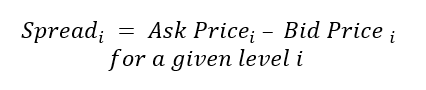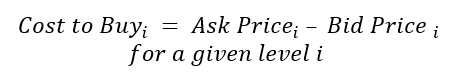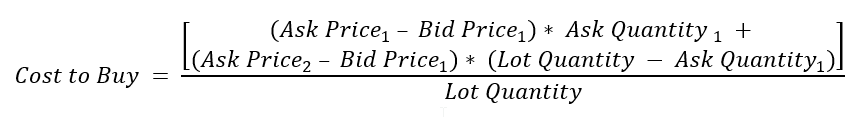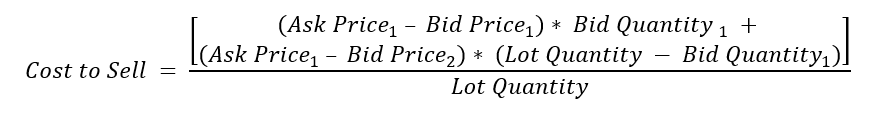# Understanding the CME Liquidity Tool Methodology

• 13 Sep 2018
• By Owain Johnson and CME Group

The CME Liquidity Tool is based on the electronic limit order book, which is constructed from CME Globex trading engine messages. The order book is updated whenever an order is created or a trade is executed or cancelled.

In the order book, each order entry is time-stamped and organized in sequence. Over each second, a time-weighted average is applied over all entries within the window. This yields a time-weighted average value for each second in time that has been aggregated from the raw order entry data.

The liquidity measures in the tool are ask price, bid price, number of bid orders, number of ask orders, bid order quantity and ask order quantity. The order book is structured for 10 levels and sorted, with ask prices in ascending order and bid prices in descending order.

The Bid-Ask Spread over each second is calculated for all levels of the order book (from 1 to 10).If either the ask price or the bid price is not available at any level of the order book, the spread will not be calculated.

Cost to trade is calculated for each second for the cost to buy and the cost to sell. This is the average cost to buy or sell a fixed lot size expressed in ticks. This is produced for all levels of the order book. The cost to trade is not calculated if there is an insufficient number of orders in the order book.

The cost to buy a fixed lot quantity at any given level is calculated as:If the lot size is not fully available at the top of the book (or level 1), then the ask price at the next levels are used in the calculation for the remaining quantity. The bid price used in the calculation remains the same irrespective of levels—the top of the book bid price. For example, if the lot size can be obtained within levels 1 and 2, the calculation is as follows:## Cost To Sell

The cost to sell is calculated in a similar manner. Note that, in this case, the ask price used in the calculation remains the same irrespective of levels — the top of the book ask price.The order book will have no entries during periods (seconds) where there is no activity. These gaps are filled by order book values from the previous second. The logic behind this is that even though there is no activity, there is still liquidity, which is the same liquidity that was present in the previous second. The gaps in the orderbook are filled only for market open times.

In order to express the order book at higher levels of granularity, the seconds-level data is aggregated to minute, hour and day levels. Each of the liquidity measures, spread, cost to trade, number of orders and order quantity, are averaged over the respective level of aggregation (minute, hour, day) from the values computed at the seconds-level. It should be noted that only market open times are used to arrive at the average.

As the world’s leading derivatives marketplace, CME Group is where the world comes to manage risk. Comprised of four exchanges - CME, CBOT, NYMEX and COMEX - we offer the widest range of global benchmark products across all major asset classes, helping businesses everywhere mitigate the myriad of risks they face in today's uncertain global economy.

Follow us for global economic and financial news.